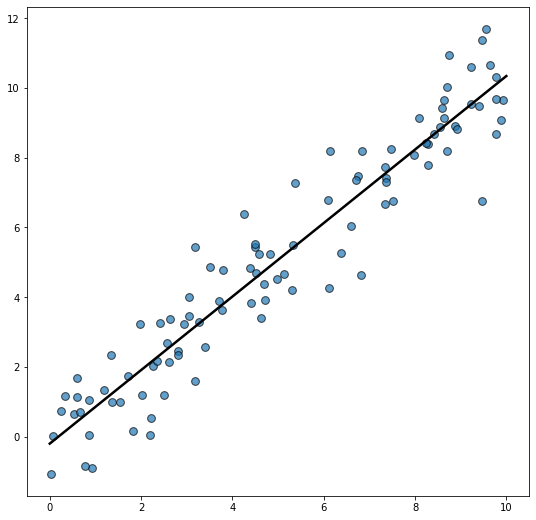Let's get started by importing Matplotlib and Numpy

``````import matplotlib.pyplot as plt
import numpy as np``````

Now let's create a reproducible random number generator. This ensures the result is the same no matter how many times we generate the random data.

``rng = np.random.default_rng(1234)``

And finally, let's generate some random data, make the scatterplot, and add the regression line:

``````# Generate data
x = rng.uniform(0, 10, size=100)
y = x + rng.normal(size=100)

# Initialize layout
fig, ax = plt.subplots(figsize = (9, 9))

ax.scatter(x, y, s=60, alpha=0.7, edgecolors="k")

# Fit linear regression via least squares with numpy.polyfit
# It returns an slope (b) and intercept (a)
# deg=1 means linear fit (i.e. polynomial of degree 1)
b, a = np.polyfit(x, y, deg=1)

# Create sequence of 100 numbers from 0 to 100
xseq = np.linspace(0, 10, num=100)

# Plot regression line
ax.plot(xseq, a + b * xseq, color="k", lw=2.5);``````## Contact & Edit

👋 This document is a work by Yan Holtz. You can contribute on github, send me a feedback on twitter or subscribe to the newsletter to know when new examples are published! 🔥